Latest Banking jobs   »

# Reasoning Ability Quiz For LIC AAO Mains 2023-18th March

Directions (1-5): Read the following information carefully and answer the following questions.
Six persons P, Q, R, S, T, and U are born in same month and date of six different year viz. 1978, 1982, 1990, 1995, 2000, and 2006 but not necessarily in the same order. (All the ages will be calculated on base year 2021). All persons are related to each other.
T is the father of the one whose age is 15 years. The difference between T’s age and P’s age is the lowest. P is the mother of U, who is a male. Q is married to the sister of P, whose age is 39 years. S is the sister-in-law of R. S is older than R but younger than Q. There are only two females in the family. U is the youngest family member.

Q1. How is S related to the one whose age is 15 years?
(a) Paternal Uncle
(b) Maternal Uncle
(C) Maternal Aunt
(d) Paternal Aunt
(e) None of these

Q2. Who was born in 1990?
(a) S
(b) R
(C) Q
(d) T
(e) Either (b) or (c)

Q3. What is the sum of the ages of S and R?
(a) 52 years
(b) 57 years
(c) 47 years
(d) 60 years
(e) None of these

Q4. Which of the following information is correct?
(a) R was born in 1995
(b) R is the brother of Q
(C) Both (a) and (b)
(d) U is the son of P and T
(e) Both (b) and (d)

Q5. Four of the following five are alike in a certain way based on a group, which of the following does not belong to that group?
(a) T- 1978
(b) P -1982
(C) Q – 1990
(d) R- 1995
(e) U- 2006

Directions (6-10): In these questions some statements are followed by some conclusions numbered. You have to take the three given statements to be true even if they seem to be at variance from commonly known facts and then decide which of the given conclusions logically follows from the given statements disregarding commonly known facts.

Q6. Statements: All hard are tough.
No tough is a question.
Some question are exam.
Conclusions: I. All hard being exam is a possibility.
II. No hard is a question.
(a) Only I follows.
(b) Only II follows.
(c) Both I and II follow.
(d) Either I or II follows.
(e) Neither I nor II follows.

Q7. Statements: All eraser are sharpener.
Only Few pencil are eraser.
No pencil is Pen
Conclusions: I. No eraser is a pen.
II. All sharpeners are pencil is a possibility.
(a) Only I follows.
(b) Only II follows.
(c) Either I or II follows.
(d) Neither I nor II follows.
(e) Both I and II follow.

Q8. Statements: All white is blue.
Some blue is olive.
No olive is a grey.
Conclusions: I. Some white is olive.
II. Some grey being blue is a possibility.
(a) Either I or II follows.
(b) Both I and II follow.
(c) Only I follows.
(d) Neither I nor II follows.
(e) Only II follows.

Q9. Statements: All earth are suns.
No sun are moon.
Few moon are Jupiter.
Conclusions: I. All earth are Jupiter.
II. No sun is a Jupiter.
(a) Either I or II follows.
(b) Only II follows.
(c) Neither I nor II follows.
(d) Only I follows.
(e) Both I and II follow.

Q10. Statements: All rectangle is square.
Some squares are circles.
No rectangle is a triangle.
Conclusions: I. All squares can never be triangle.
II. At least some rectangle is circle.
(a) Either I or II follows.
(b) Both I and II follow.
(c) Neither I nor II follows.
(d) Only I follow.
(e) Only II follows.

Directions (11-15): Study the information carefully and answer the questions given below.
“Quad Core processor clocks” is written as ‘&5Z &5V \$9V &8L’
“Massive internal memory” is written as ‘\$7Z &8Z &7V’
“Which makes multitasking” is written as ‘\$7R \$6Z &11Z’

Q11. What is the code for ‘Snapdragon’ in the given code language?
(a) &10L
(b) \$10Z
(c) \$ 7Z
(d) &10Z
(e) None of these

Q12. What is the code for ‘shade owners’ in the given code language?
(a) \$6Z \$7V
(b) \$6Z &7V
(c) &6Z \$7V
(d) Can’t be determined
(e) None of these.

Q13. What does the code ‘&8R’ stand for in the given code language?
(a) yellow
(b) in
(c) blue
(d) Bright
(e) None of these

Q14. What is the code for ‘blue heaven love’ in the given code language?
(a) &5V &6Z &5V
(b) \$5V &8Z \$5V
(c) &8Z \$5V \$5V
(d) Can’t be determined
(e) None of these

Q15. ‘&8L &6L &5V’ is the code for which of the following?
(a) Cloths colour blue
(c) Is in blue
(d) Can’t be determined
(e) None of these

Solutions

Solutions (1-5):
Sol. By using the given information: (All the ages will be calculated on base year 2021). So, the age of persons will be 43 years, 39 years, 31 years, 26 years, 21 years, and 15 years.
First, we try to determine the blood relations:
U is the youngest family member. T is the father of the one whose age is 15 years. Q is married to the sister of P, whose age is 39 years. The difference between T’s age and P’s age is the lowest so it means T age is 43 years. S is the sister-in-law of R. P is the mother of U, who is a male, so P and T are parents of U, whose age is 15 years.
Q is married to the sister of P, whose age is 39 years. S is the sister-in-law of R. There are only two females in the family. Now we can get all the blood relations between the persons.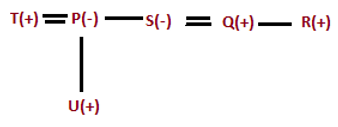The difference between T’s age and P’s age is the lowest, So P’s age is 39 years and T’s age is 43 years and U’s age is 15 years. S is older than R but younger than Q. Now we have our final arrangements.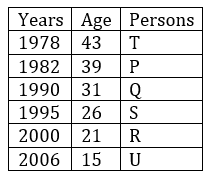S1. Ans. (c)
S2. Ans. (c)
S3. Ans. (c)
S4. Ans. (e)
S5. Ans. (d)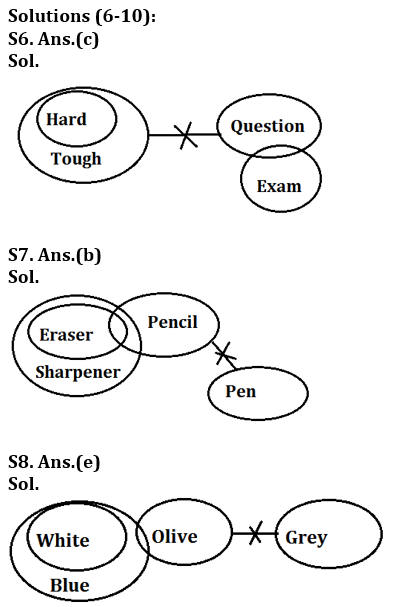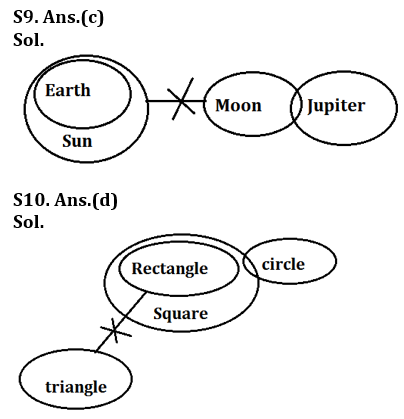Solutions(11-15):
S11. Ans.(d)
Sol. Logic: – 1st element of the code: – If the word has odd number of letters, then we use \$ symbol. If the word has even number of letters in the word, then we use & symbol.
2nd element of the code:- Add 3 in total number of consonant present in the word.
3rd element of the code:- Opposite of vowel which comes 1st according to increasing alphabetical order among all the vowels present in the word.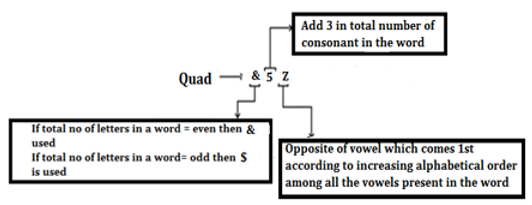S12. Ans.(b)
Sol. Logic: – 1st element of the code:- If the word has odd number of letters then we use \$ symbol. If the word has even number of letters in the word then we use & symbol.
2nd element of the code:- Add 3 in total number of consonant present in the word.
3rd element of the code:- Opposite of vowel which comes 1st according to increasing alphabetical order among all the vowels present in the word.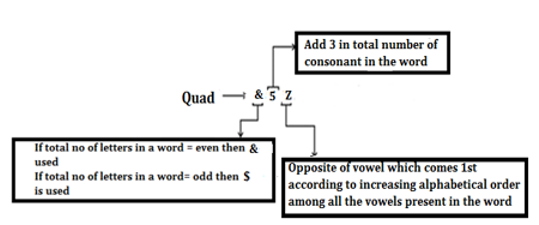S13. Ans.(d)
Sol. Logic: – 1st element of the code:- If the word has odd number of letters then we use \$ symbol. If the word has even number of letters in the word then we use & symbol.
2nd element of the code:- Add 3 in total number of consonant present in the word.
3rd element of the code:- Opposite of vowel which comes 1st according to increasing alphabetical order among all the vowels present in the word.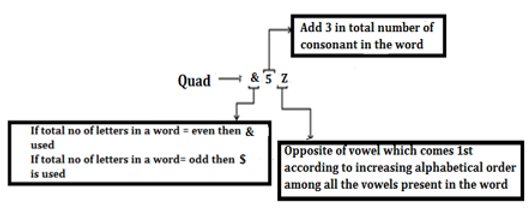S14. Ans.(a)
Sol. Logic: – 1st element of the code:- If the word has odd number of letters then we use \$ symbol. If the word has even number of letters in the word then we use & symbol.
2nd element of the code:- Add 3 in total number of consonant present in the word.
3rd element of the code:- Opposite of vowel which comes 1st according to increasing alphabetical order among all the vowels present in the word.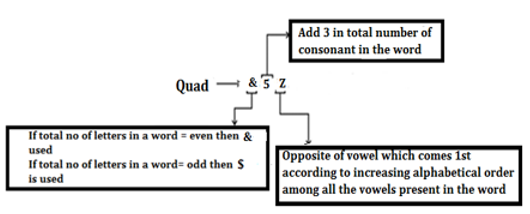S15. Ans.(a)
Sol. Logic: – 1st element of the code:- If the word has odd number of letters then we use \$ symbol. If the word has even number of letters in the word then we use & symbol.
2nd element of the code:- Add 3 in total number of consonant present in the word.
3rd element of the code:- Opposite of vowel which comes 1st according to increasing alphabetical order among all the vowels present in the word.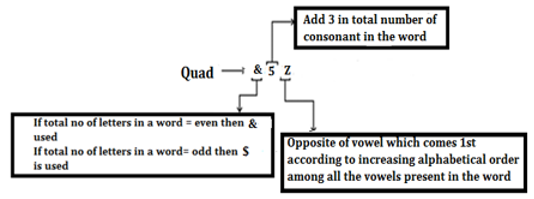## FAQs

### When will the LIC AAO Mains exam be held?

LIC AAO Mains exam will be held on 18 March

#### Congratulations!Union Budget 2023-24: Free PDF